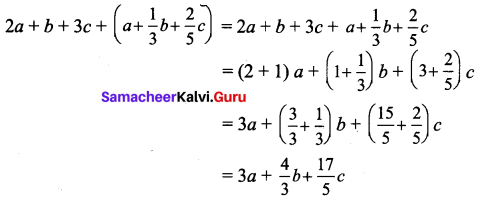# Samacheer Kalvi 7th Maths Solutions Term 1 Chapter 3 Algebra Ex 3.4

Students can Download Maths Chapter 3 Algebra Ex 3.4 Questions and Answers, Notes Pdf, Samacheer Kalvi 7th Maths Book Solutions Guide Pdf helps you to revise the complete Tamilnadu State Board New Syllabus and score more marks in your examinations.

## Tamilnadu Samacheer Kalvi 7th Maths Solutions Term 1 Chapter 3 Algebra Ex 3.4

Miscellaneous Practice Problems

Question 1.
Subtract – 3ab – 8 from 3ab – 8. Also subtract 3ab + 8 from -3ab – 8.
Solution:
Subtracting -3ab – 8 from 3ab + 8
= 3ab + 8 – (-3ab – 8) = 3ab + 8 + (3ab + 8)
= 3ab + 8 + 3ab + 8 = (3 + 3) ab + (8 + 8)
= 6ab + 16
Also subtracting 3 ab + 8 from – 3ab – 8
= – 3ab – 8 – (3ab + 8) = – 3ab – 8 + (-3ab – 8) = – 3ab – 8 – 3 ab – 8
= [(-3) + (- 3)] ab + [(-8) + (-8)] = – 6ab + (- 16)
= -6ab – 16

Question 2.
Find the perimeter of a triangle whose sides are x + 3y, 2x + y, x – y.
Solution:
Perimeter of a triangle = Sum of three sides
= (x + 3y) + (2x + y) + (x – y)
= x + 3y + 2x + y + x – y
= (1 + 2 + 1)x + (3 + 1 + (-1))y = 4x + 3y
∴ Perimeter of the triangle = 4x + 3y

Question 3.
Thrice a number when increased by 5 gives 44. Find the number.
Solution:
Let the required number be x.
Thrice the number = 3x.
Thrice the number increased by 4 = 3x + 5
Given 3x + 5 = 44
3x + 5 – 5 = 44 – 5
3x = 39
$$\frac{3 x}{3}=\frac{39}{3}$$
x = 13
∴ The required number = 13

Question 4.
How much smaller is 2ab + 4b – c than 5ab – 3b + 2c.
Solution:
To find the answer we have to find the difference.
Here greater number 5ab – 3ab + 2c.
∴ Difference = 5ab – 3b + 2c – (2ab + 4b – c) = 5ab – 3b + 2c + (- 2ab -4b + c)
= 5ab – 3b + 2c – 2ab – 4b + c
= (5 – 2) ab + (-3 – 4) b + (2 + 1) c = 3ab + (-7)b + 3c
= 3ab – 7b + 3c
It is 3ab – 7b + 3c smaller.Question 5.
Six times a number subtracted from 40 gives – 8. Find the number.
Solution:
Let the required number be x. Six times the number = 6x.
Given 40 – 6x = – 8
-6x + 40 – 40 = -8 – 40
– 6x = – 48
$$\frac{-6 x}{-6}=\frac{-48}{-6}$$
x = 8
∴ The required number is 8.

Challenge Problems

Question 6.
From the sum of 5x + 7y -12 and 3x – 5y + 2, subtract the sum of 2x – 7y – 1 and – 6x + 3y + 9.
Solution:
Sum of 5x + 7y – 12 and 3x – 5y + 2 .
= 5x + 7y- 12 + 3x – 5y + 2 = (5 + 3) x + (7 – 5) y + ((- 12) + 2)
= 8x + 2y – 10.
Again Sum of 2x – 7y – 1 and – 6x + 3y + 9
= 2x – 7y – 1 + (- 6x + 3y + 9) = 2x – 7y – 1 – 6x + 3y + 9
= (2 – 6) x + (- 7 + 3) y + (- 1 + 9)
= – 4x – 4y + 8
Now 8x + 2y – 10 – (-4x – 4y + 8)
= 8x + 2y – 10 + (4x + 4y – 8)
= 8x + 2y – 10 + 4x + 4y – 8
= (8 + 4) x + (2 + 4) y + ((- 10) + (- 8))
= 12x + 6y – 18

Question 7.
Find the expression to be added with 5a – 3b – 2c to get a – 4b – 2c?
Solution:
To get the required expression we must subtract 5a – 3b + 2c from a – 4b – 2c.
∴ a – 4b – 2c – (5a – 3b + 2c) = a – 4b – 2c + (- 5a + 3b – 2c)
= a – 4b – 2c – 5a + 3b -2c
= (1 – 5) a + (- 4 + 3) b + (- 2 – 2) c
= – 4a – b – 4c.
∴ -4a – b – 4c must be added.

Question 8.
What should be subtracted from 2m + 8n + 10 to get – 3m + 7n + 16?
Solution:
To get the expression we have to subtract – 3m + 7n + 16 from 2m + 8n + 10.
(2m + 8n + 10) – (-3m + 7n + 16) = 2m + 8n + 10 + 3m – 7n – 16
= (2 + 3) m + (8 – 7) n + (10 – 16)
= 5m + n – 6Question 9.
Give an algebraic equation for the following statement:
“The difference between the area and perimeter of a rectangle is 20”.
Solution:
Let the length of a rectangle = l and breadth = b then Area = lb; Perimeter = 2(1 + b)
Area – Perimeter = 20
∴ lb – 2(l + b)

Question 10.
Add : 2a + b + 3c and a + $$\frac{1}{3}$$b + $$\frac{2}{5}$$c
Solution: